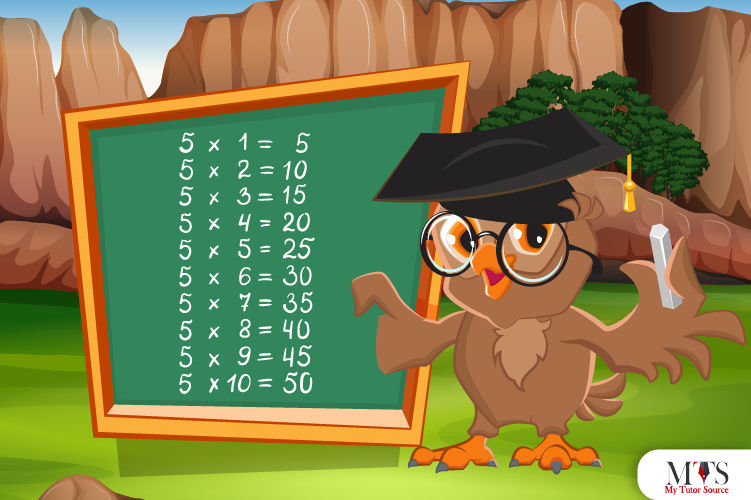# Multiplication Table of 5 – Tips to Memorize 5 Times Table & Example QuestionsLearning lower times tables could be challenging for students, but 5 times table is a breeze. Math tutors and early elementary school teachers ensure their students memorize multiplication tables up to 10 by heart. The pattern of multiplication table 5 is so easy that some tutors or parents let their children learn it after table 2. You can use the same strategy on your child as the property of a 5 times table is relatively easy and obvious. Give this strategy a try. it will definitely work. Furthermore, give this article a read and learn table of 5 and tips to memorize and practice given example questions.

## Table of 5

For division, long multiplication, fraction, and other arithmetic queries, the knowledge of 5 times tables counts. It’s helpful to understand the working of the clock better. Below we have given the table of 5 till 20. try memorizing it into parts.

5 x 1 = 5
5 x 2 = 10
5 x 3 = 15
5 x 4 = 20
5 x 5 = 25
5 x 6 = 30
5 x 7 = 35
5 x 8 = 40
5 x 9 = 45
5 x 10 = 50
5 x 11 = 55
5 x 12 = 60
5 x 13 = 65
5 x 14 = 70
5 x 15 = 75
5 x 16 = 80
5 x 17 = 85
5 x 18 = 90
5 x 19 = 95
5 x 20 = 100

## Method to Read Table of 5

In this section, you will have a closer look at the multiplication table of 5. Rather than struggling with the table, read the table given in words below. It will help you a lot in memorizing:

One time five is five (5)
Two times five is ten (10)
Three times five is fifteen (15)
Four times five is twenty (20)
Five times five is twenty-five (25)
Six times five is thirty (30)
Seven times five is thirty-five (35)
Eight times five is forty (40)
Nine times five is forty-five (45)
Ten times five is fifty (50)
Eleven times five is fifty-five (55)
Twelve times five is sixty (60)
Thirteen times five is sixty-five (65)
Fourteen times five is seventy (70)
Fifteen times five is seventy-five (75)
Sixteen times five is eighty (80)
Seventeen times five is eighty-five (85)
Eighteen times five is ninety (90)
Nineteen times five is ninety-five (95)
Twenty times five is hundred (100)

## Tips for Memorizing Multiplication Table of 5

Here are a few quick tips for memorizing the 5 times tables quickly and easily:

1. Follow the pattern. The unit place of the 5 times table’s product will always be 5 or 0.
2. Count in 5s to get the answers of 5 times table’s multipliers. Kids can also use building blocks, objects, or legos to understand addition and product rules.
3. Listen to five times table songs. Human brains catch the rhythm faster than other terms. For instance, the rhythm of five, ten, fifteen, twenty, twenty-five, thirty, thirty-five, forty, forty-five, and fifty seems easy to understand and learn. Therefore, reciting it repeatedly would definitely work.
4. Play a Hopscotch game with blocks filled with products of the table of 5. Let the child hop onto the product of 5 and learn simultaneously.

### Example Question of Table of 5

Question: Diya rides her bike around 5 miles per day. If she will ride the same miles for 2 weeks, how many miles must she cover in a given period?

Solution:

Given that,
Number of miles per day = 5 miles
Total days = 2 weeks = 14 days
Therefore, using the 5 times table, the number of miles covered by Diya within 2 weeks = 5 x 14 = 70
Hence, Diya would ride her bike 70 miles within 2 weeks.

Question: 5 roses in a basket. How many roses are in 8 baskets?

Solution:

5 x 8 = 40
Thus, 8 baskets will have 40 roses.

Question: What is eleven times five?

Solution:

11 x 5 = 55
Hence, eleven times 5 is equal to fifty-five.

Question: What are 5 times table up to 10?

The 5 times table up to 10 is as follows:
5 x 1 = 5
5 x 2 = 10
5 x 3 = 15
5 x 4 = 20
5 x 5 = 25
5 x 6 = 30
5 x 7 = 35
5 x 8 = 40
5 x 9 = 45
5 x 10 = 50

Question: Find out the value of 5 times 5 plus 5?

Solution:

Write 5 times 5 plus 5 in mathematically form
5 times 5 plus 5 = 5 x 5 + 5 = 25 + 5 = 30
Hence, 5 times 5 plus 5 is equal to 30.

### Find Top Tutors in Your AreaWith over 3 years of experience in teaching, Chloe is very deeply connected with the topics that talk about the educational and general aspects of a student's life. Her writing has been very helpful for students to gain a better understanding of their academics and personal well-being. I’m also open to any suggestions that you might have! Please reach out to me at chloedaniel402 [at] gmail.com